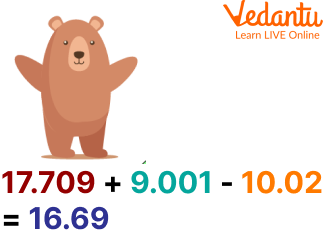Courses
Courses for Kids
Free study material
Free LIVE classes
More

# Simplification of Decimal NumbersLIVE
Join Vedantu’s FREE Mastercalss

## Simplification of Decimal Numbers: An Introduction

Have you ever thought about the use of decimal numbers? Decimal numbers are used to denote the numerical quantity that is not the whole but in fractions. For example, 2.35, 1.2, 5.67, etc. all denote decimal numbers. After reading this article, students will be able to understand the simplification of decimal numbers. This is the most basic and interesting topic of Mathematics used in everyday life. Let us now start with the conceptual clarification of the decimals.

## What is Decimal Simplification?

Decimal represents a number with decimal points. Simplifying a decimal point means rewriting the like terms of an expression together and solving them by using the BODMAS rule and some decimal simplification tricks.

## Decimal Simplification Tricks

Some of the decimal simplification tricks are given below:

• Put all the likes terms together and solve them.

• Put in the BODMAS rule.

• For convenience, convert the decimal point to a fraction and then solve further.

• After the solution, convert the fraction back to the decimal point.

## Rules of Decimal Simplification

Simplification of decimal numbers involves a series of rules to be followed:

$V \rightarrow V$ stands for Vinculum.

$B \rightarrow B$ stands for Removing Brackets.

Removal of bracket takes place in the $\operatorname{order}(),\{\},[]$.

$O \rightarrow O$ stands for of.

$D \rightarrow$ D stands for Division.

$\mathrm{M} \rightarrow \mathrm{M}$ stands for Multiplication.

$A \rightarrow$ A stands for Addition.

$\mathrm{S} \rightarrow \mathrm{S}$ stands for Subtraction.

## Solved Examples

1. Simplify 17.709 + 9.001 - 10.02.

Ans: Simplification of decimals in the expression is given by the following steps:

• Write the given decimal expression

17.709 + 9.001 - 10.02

• Put in the BODMAS rule to the given expression

26.710 - 10.02

• Solving the expression further, we get,

16.69

Hence, the simplification of the decimal expression is 16.69.Simplification of Decimal Expression

2. Solve $2.8 \times 10+4.2-2.9+4.5 \div 5$.

Ans: Simplification of decimals in the expression is given by the following steps:

Step 1: Write the given decimal expression.

$2.8 \times 10+4.2-2.9+4.5 \div 5$

Step 2: Put in the BODMAS rule to the given expression.

$=2.8 \times 10+4.2-2.9+0.9$

$=28+4.2-2.9+0.9$

Step 3: Solving the expression further, we get,

$=32.2-2.0$

$=30.2$

Hence, the simplification of decimal numbers is $30.2$.

## Practice Problems

1. Simplify 17.79 - 19.01 + 11.02.

Ans: 9.80

2. Simplify the given decimal:

$5+2.4 \div (3.1-0.7)$

Ans: $6$

3. Calculate $\left[\frac{(5.39)^2-(2.91)^2}{(5.39)-(2.91)}\right]$.

Ans: 8.3

## Decimal Simplification Worksheets

1) $4.25 \times 1.4+9$

2) $92.7 \div 3 \times 25+0.17$

3) $19+6.14 \times 3.5+6$

4) $81-0.96 \div 12$

5) $7.2 \div 0.08+15.2$

6) $2.4 \times 0.3+36-5.6$

7) $42-3.4 \times 7.5-0.18$

8) $213-2.12 \times 8.5$

9) $1.6 \times 9.3-\mathrm{t} 5$

10) $9.9 \div 3 \times 1.5 \div 13.4$

## Summary

Finishing up here with the concept of decimal simplification. It is a basic topic of Maths that has wide applications in everyday life. This article has covered decimal simplification tricks, worksheets and solved examples for better clarification of the concepts. The language used in the above article is very simple and to the point. Some practice problems are given above that need to be solved by the students themselves to master the topic deeply. we hope that you enjoyed reading the article.

Last updated date: 19th Sep 2023
Total views: 93k
Views today: 0.93k

## FAQs on Simplification of Decimal Numbers

1. Is the decimal and fraction of a number the same thing?

No, the decimal and the fraction are not the same things. The two depict different notations to represent a number. The decimal is used to write the number in two parts by separating each part with a decimal point i.e. dot while the fraction is used to represent a number in the form of $\dfrac{p}{q}$. Both the notations used to represent a number are interconvertible i.e. decimals can be changed to fractions and vice versa.

2. What is the use of decimal simplification pdf?

Decimal simplification pdf serves as a useful tool, available offline, for grasping the concepts deeply. It is used to understand the way of solving the problem based on decimals. Decimal simplification pdf can easily be downloaded from the internet.

3. How many types of decimal numbers are there?

There are two different types of decimal numbers:

• Terminating Decimal Numbers: A decimal number,  which has a fixed number of digits after the decimal point is called a terminating decimal number.

• Non-terminating Decimal Numbers: A decimal number which has an infinite number of digits after the decimal point is called a non-terminating decimal number.